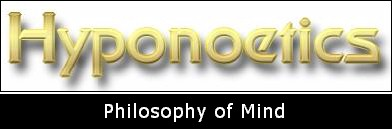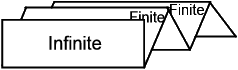This blog discusses ideas and concepts that I am currently thinking about for my book on Hyponoetics as an integral philosophy of mind and matter.

### The Infinite and the Finite

Mar 01, 2023 - Category: Metaphysics  Ontology - Tag: Exonoesis  Hyponoesis  Infinite  Paranoesis  Reality

The relationship between the Infinite and the Finite is one of the basic metaphysical principles discussed by philosophers. The Infinite does not refer to a mathematical infinite, but to an entity that is boundless, without any limitations of space and time.

To be finite is to be a thing that is limited by another thing. A finite thing possesses a limited number of properties which define that thing as different from another thing with a similar or different set of properties. Whatever has boundaries is finite. Whatever we perceive through our senses is finite, otherwise we would not be able to distinguish one thing from another. The finite exists in space and time which are the two most essential properties of differentiation.

The Infinite, per definitionem, is what does not have any limitations and therefore no finite set of properties. The Infinite is not so much an 'object' of perception or thought, but rather a metaphysical idea that extends our rational thinking to a point where everything becomes one: the identity of all differentiations and of all opposites. The Infinite contains potentially all the finite forms and each finite form is potentially the Infinite. This is the dictum of the German idealist philosophers and also plays an important part in Hyponoetics.

The Pre-Socratic philosopher Anaximander (ca. 611-546 BC) was probably the first thinker who contemplated the concept of the Infinite. He thought of the Infinite (τὸ ἅπειρος, to apeiros) as a basic, unlimited substance of which everything that exists, i.e. the limited things, consists. It is an eternal, inexhaustible, and indeterminate substance.

Later the Pythagoreans adopted this idea of the Unlimited and introduced a limiting principle (πέρας, peras), which manifests the Infinite as a finite form. Similarly, Plato maintained that both the limited and the unlimited are two principles of being that together structure the world.

Plotinus (ca. 204-270) applied the concept of the Infinite to both matter and mind. Matter is infinite because it is intrinsically formless. Mind is infinite because it has endless power and represents a complete, self-sufficient unity. Both mind and matter emanated (emerged) from the One: "Absolutely One, it has never known measure and stands outside of number, and so is under so limit either in regard to anything external or internal; for any such determination would bring something of the dual into it." [Enneads, V.5.11].

The Scholastic philosophers associated the idea of the Infinite with God. The world is the finite creation of the infinite power of God. God's infinity is expressed in the Scholastic dictum of the identity of essence and existence in God as opposed to the created thing in which a thing’s essence limits its existence.

Georg Cantor was the first to create a mathematical theory of the infinite: "The actual infinite arises in three contexts: first when it is realized in the most complete form, in a fully independent other-worldly being, in Deo [God], where I call it the Absolute Infinite or simply Absolute; second when it occurs in the contingent, created world; third when the mind grasps it in abstracto as a mathematical magnitude, number, or order type. I wish to make a sharp contrast between the Absolute and what I call the Transfinite, that is, the actual infinities of the last two sorts, which are clearly limited, subject to further increase, and thus related to the finite." [Gesammelte Abhandlungen, p. 378, Springer Verlag, 1932].

The problem that the German Idealists Schelling and Hegel recognized was that if the Infinite is distinct from the Finite, then the finite, formed thing is limited by the Infinite and, therefore, itself becomes finite. Thus, they believed that ultimately, the Finite is not distinct from the Infinite but is just an aspect or moment of the Infinite. The differences of the Finite are canceled or sublated in the synthesis or identity-in-differences of the Infinite or Absolute.

Nicholas of Cusa (1401-64) postulated a similar idea when he proposed a 'synthesis of opposites' (coincidentia oppositorum). He thought that opposites no longer exist in the concept of the Infinite. Finite and limited things have opposites because they allow to have more or less of something. The Infinite does not have more or less, otherwise it would be limited. Therefore, opposites coincide in the Infinite.

Hyponoetics' concept of the Infinite is similar to the German Idealists. Hyponoesis is the ultimate reality that is an identity of the Infinite and the Finite. What then is the Finite? It is the manifestation or projection of the Infinite. The Finite is the result of the self-projection of the Infinite into a plurality of limited and individual forms and structures.

If the Finite is the self-projection of the Infinite, then, reciprocally, the Infinite is the self-reflection of the Finite. Projection is derived from the Latin term proiecere meaning to throw forward. Reflection is derived from Latin reflectere meaning to bend back, to turn back. By self-reflection, therefore, I mean the process of the Individual Mind (Exonoesis) of expanding its mind into the Infinite by turning all its attention to itself. It is a process of de-individuation, that is, by reflecting on the mind's intrinsic infinity, the Individual Mind looses its individuality temporarily and is able to expand into the Infinite Mind (Hyponoesis). I call this process Paranoesis or Transrational Thinking.

It is important to understand that the process of self-projection of the Infinite, that manifests the finite world, is not a process of creation. The world and all living creatures are not created, neither ex nihilo (out of nothing) as Christian theology holds, nor in any other way. The Infinite actualizes itself in an infinite number of forms without creating them as something distinct from itself.

We could also think of the Infinite and the Finite as congruent dimensions or continua of one reality. The infinite continuum subsists as any number of finite continua which are congruent and simultaneous. If we only think about the infinite continuum then its infinity consists in having an infinite number of potential finite continua. If we only consider one specific finite continuum, its finiteness consists in being limited and distinct from another finite continuum.

Here's a way to visually present this rather complex idea: Take a piece of paper (letter size). Fold it widthwise across several times into smaller sections with identical size:Figure 2 - Model illustrating the relationship between the Infinite and the Finite

On the front fold write: "Infinite". On the subsequent folds write: "Finite". If the whole paper is folded together to form one piece and you look at it when you have it at eye level, then you can only see the label "Infinite". If you unfold the paper and slightly tilt the back end upwards so as to reveal the other folds with the labels "Finite", then you can see, how the Infinite is "made up" of the Finite, and how both together constitute one inseparable and timeless entity. This is a very crude way of making the concept of the Identity of the Infinite and the Finite a little bit more intelligible.